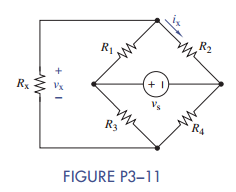Chat Now

# Formulate node-voltage equations for the circuit in Figure P3−11. (b) Solve for v x and ix..

### Formulate node-voltage equations for the circuit in Figure P3−11. (b) Solve for v x and ix..

(a) Formulate node-voltage equations for the circuit in Figure P3−11.

(b) Solve for vx and ix when R1 =1kΩ, R2 = 1:5 kΩ, R3 = 500 Ω, R4 =2kΩ, Rx = 100 Ω, and v= 15 V.manish jayant 28-Jul-2020Get solution